### Home > CCA > Chapter 9 > Lesson 9.2.2 > Problem9-59

9-59.
1. Solve the inequalities below for the given variables. Represent your solutions on a number line. 9-59 HW eTool (CPM). Homework Help ✎

1. 3(2k − 1) < 9

2.≤ 6

3. −2 + 8n > 2

4. 7t − 4 > 2t − 4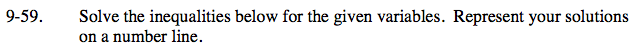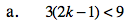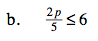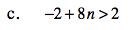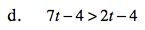Solve as an equation for k.

Distribute the left side.
6k − 3 < 9

Add 3 to both sides.
6k < 12

Divide by 6 on both sides.
k < 2

Test k = 0
6(0) − 3 < 9
−3 < 9

Solve as an equation for p.

Multiply both sides by 5.
2p ≤ 30

Divide both sides by 2.
p ≤ 15

Test p = 0
2(0) ≤ 30
0 ≤ 30

See the hints in parts (a) and (b).

See the hints in parts (a) and (b).

Use the eTool below to complete the number lines.
Click the link at right for the full version of the eTool: CCA 9-59 HW eTool